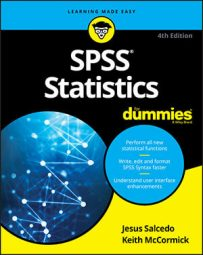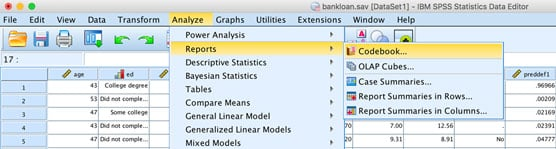##### SPSS Statistics For DummiesIBM SPSS Statistics is an application that performs statistical analysis on data. To perform statistical analyses correctly, you need to know the level of measurement of the variables because it defines which summary statistics and graphs should be used. It also helps to know the most commonly used procedures in the Analyze menu and possible conclusions that you can reach after conducting a statistical test.SPSS Statistics Analyze menu

## SPSS statistics variables level of measurement

Level of measurement defines which summary statistics and graphs should be used. The following table provides definitions, examples, appropriate summary statistics, and graphs for variables based on their level of measurement.

Nominal Ordinal Scale
Definition Unordered categories Ordered categories Both interval and ratio
Examples Gender, geographic location, job category Satisfaction ratings, income groups, ranking of
preferences
Number of purchases, cholesterol level, age
Measures of Central Tendency Mode Median Median or mean
Measures of Dispersion None Min/max/range Min/max/range, Standard deviation/ variance
Graph Pie or bar Bar Histogram

## SPSS statistics chart to show relationships between a pair of variables

When choosing a graph, you need to know the level of measurement of the variables. The following table shows some of the graphs that can be used to display relationships between different types of variables.

Categorical Dependent Scale Dependent
Categorical Independent Clustered bar or paneled pie Error bar or boxplot
Scale Independent Error bar or boxplot Scatter plot

## SPSS statistics commonly used analyze menus

The following table provides a list of some of the most commonly used procedures in the Analyze menu in SPSS Statistics.

 Menu Submenu Useful For Code Book Reports Provides a quick look at all your variables at once. The level of measurement automatically controls which summary statistics are displayed. Frequencies Descriptives Tells you how many of each category value you have. Most useful for categorical variables because you can run all of them at once. Descriptives Descriptives Gets basic scale variable information, such as the mean and standard deviation. Explore Descriptives Based on a famous book, Exploratory Data Analysis, looks at all kinds of variables as well as pairs of variables. Crosstabs Descriptives Tests to see if categorical variables are independent of each other or related to each other. Means Compare Means Calculates subgroup means and related statistics for dependent variables within categories of one or more independent variables. One-Sample T-Test Compare Means Tests whether the mean of a single variable differs from a specified value (for example, a group using a new learning method compared to the school average). Independent Samples T-Test Compare Means Tests whether the means for two groups differ on a continuous dependent variable (for example, females versus males on income). Paired Samples T-Test Compare Means Tests whether a significant difference exists in the mean under two conditions (for example, before versus after, or standing versus sitting). One-Way ANOVA Compare Means Tests whether the means for two or more groups differ on a continuous dependent variable (for example, drug1 versus drug2 versus drug3 on depression). Bivariate Correlation Correlate Determines the similarity in the way two continuous variables change in value from one case (row) to another through the data. Linear Regression Regression Predicts a continuous dependent variable from one or more continuous independent variables One Sample Nonparametric Tests Compares the distribution of a categorical dependent variable to population norms. Independent Samples Nonparametric Tests Tests whether the means or medians for two or more different groups differ on a dependent variable. Related Samples Nonparametric Tests Tests whether the means or medians of the same group differ under two conditions or time points. Univariate General Linear Model An extension of one-way ANOVA in which there is more than one independent variable. Multivariate General Linear Model An extension of one-way ANOVA in which there is more than one dependent variable. Repeated Measures General Linear Model An extension of the paired-samples t-test in which the same group is assessed under two or more conditions or time points. Binary Logistic Regression Used in situations similar to linear regression but the dependent variable is dichotomous. Multinomial Logistic Regression An extension of binary logistic regression in which the dependent variable is not restricted to two categories. Discriminant Classify Builds a predictive model for group membership based on the linear combinations of predictors that best separate the groups.

## Interpreting statistical significance in SPSS statistics

When conducting a statistical test, too often people jump to the conclusion that a finding “is statistically significant” or “is not statistically significant.” Although that is literally true, it doesn’t imply that only two conclusions can be drawn about a finding.

What if in the real world no relationship exists between the variables, but the test found that there was a significant relationship? In this case, you would be making a false positive error because you falsely concluded a positive result (you thought it does occur when in fact it does not).

On the other hand, what if in the real world a relationship does exist between the variables, but the test found that there was no significant relationship? In this case, you would be making a false negative error, because you falsely concluded a negative result (you thought it does not occur when in fact it does).

In the Real World Statistical Test Results
Not Significant (p > 0.5) Significant (p < 0.5)
The two groups are not different The null hypothesis appears true, so you conclude the groups
are not significantly different.
False positive.
The two groups are different False negative. The null hypothesis appears false, so you conclude that the
groups are significantly different.